SSC CGL Previous Year Questions: Ratio, Proportion, Mixture and Partnership - 1

# SSC CGL Previous Year Questions: Ratio, Proportion, Mixture and Partnership - 1 - SSC CGL

Test Description

## 20 Questions MCQ Test SSC CGL (Tier - 1) - Previous Year Papers (Topic Wise) - SSC CGL Previous Year Questions: Ratio, Proportion, Mixture and Partnership - 1

SSC CGL Previous Year Questions: Ratio, Proportion, Mixture and Partnership - 1 for SSC CGL 2023 is part of SSC CGL (Tier - 1) - Previous Year Papers (Topic Wise) preparation. The SSC CGL Previous Year Questions: Ratio, Proportion, Mixture and Partnership - 1 questions and answers have been prepared according to the SSC CGL exam syllabus.The SSC CGL Previous Year Questions: Ratio, Proportion, Mixture and Partnership - 1 MCQs are made for SSC CGL 2023 Exam. Find important definitions, questions, notes, meanings, examples, exercises, MCQs and online tests for SSC CGL Previous Year Questions: Ratio, Proportion, Mixture and Partnership - 1 below.
Solutions of SSC CGL Previous Year Questions: Ratio, Proportion, Mixture and Partnership - 1 questions in English are available as part of our SSC CGL (Tier - 1) - Previous Year Papers (Topic Wise) for SSC CGL & SSC CGL Previous Year Questions: Ratio, Proportion, Mixture and Partnership - 1 solutions in Hindi for SSC CGL (Tier - 1) - Previous Year Papers (Topic Wise) course. Download more important topics, notes, lectures and mock test series for SSC CGL Exam by signing up for free. Attempt SSC CGL Previous Year Questions: Ratio, Proportion, Mixture and Partnership - 1 | 20 questions in 20 minutes | Mock test for SSC CGL preparation | Free important questions MCQ to study SSC CGL (Tier - 1) - Previous Year Papers (Topic Wise) for SSC CGL Exam | Download free PDF with solutions
 1 Crore+ students have signed up on EduRev. Have you?
SSC CGL Previous Year Questions: Ratio, Proportion, Mixture and Partnership - 1 - Question 1

### A milkman uses three containers for selling milk, their capacities being 40 L, 30 L and 20 L respectively. He fills respectively 87.5%, 80% and 90% of the containers with a mix of milk and water in the ratios, 3 : 2, : 5 : 1 and 7 : 2 respectively. What is the ratio of the total quantity of milk to that of water carried by him?    (SSC CHSL-2018)

Detailed Solution for SSC CGL Previous Year Questions: Ratio, Proportion, Mixture and Partnership - 1 - Question 1

Quantity in container of capacity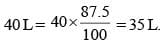Quantity in container of capacity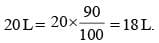Milk in three Containers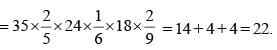Ratio of milk to water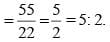SSC CGL Previous Year Questions: Ratio, Proportion, Mixture and Partnership - 1 - Question 2

### What is the ratio of the mean proportional between 8.1 and 3.6 and the third proportional of 2 and 3?    (SSC Sub-Inspector-2018 )

Detailed Solution for SSC CGL Previous Year Questions: Ratio, Proportion, Mixture and Partnership - 1 - Question 2

Mean proportion of 8.1 and 3.6 = 11.7/2 = 5.8
and third proportion of 2 and 3 is = 3/0.66 = 4.5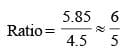SSC CGL Previous Year Questions: Ratio, Proportion, Mixture and Partnership - 1 - Question 3

### Raman, Manan, and Kamal are partners and invest in a business such that Raman invests 2/5th of total and Manan invest 3/8th of the total. What is the ratio of profit of Raman, Manan and Kamal respectively?    (SSC Sub. Ins. 2017)

Detailed Solution for SSC CGL Previous Year Questions: Ratio, Proportion, Mixture and Partnership - 1 - Question 3

Let total investment = x
Investment by Raman = 2x / 5
Investment by Manan = 3x / 8
Investment by Kamal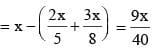Ratio of invest of Raman, Manan and Kamal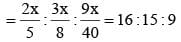∴ Ratio of profit of Raman, Manan and Kamal
= 16 : 15 : 9

SSC CGL Previous Year Questions: Ratio, Proportion, Mixture and Partnership - 1 - Question 4

If 2A = 3B and 3B = 2C, then what is A : B : C?    (SSC Sub. Ins. 2017)

Detailed Solution for SSC CGL Previous Year Questions: Ratio, Proportion, Mixture and Partnership - 1 - Question 4

Here,
2A = 3B and 3B = 2C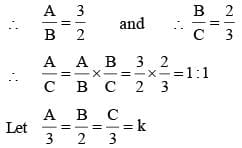Then A = 3k,  B = 2k,  C = 3k,
∴ A : B : C = 3 : 2 : 3

SSC CGL Previous Year Questions: Ratio, Proportion, Mixture and Partnership - 1 - Question 5

A litre of water weighs a kilogram, a litre of an other liquid weighs 1.340 kilograms. A mixture of the two weighs 1.270 kilograms per litre. The ratio of their volumes in a litre of the mixture is:    (SSC MTS 2017)

Detailed Solution for SSC CGL Previous Year Questions: Ratio, Proportion, Mixture and Partnership - 1 - Question 5

According to the question,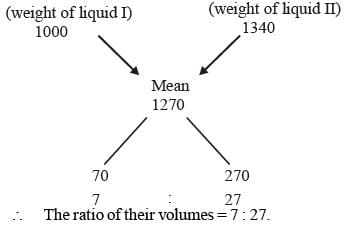SSC CGL Previous Year Questions: Ratio, Proportion, Mixture and Partnership - 1 - Question 6

Of the three numbers, the first is twice the second, and the second is twice the third. The average of the reciprocal of the numbers is 7/12. The numbers are:    (SSC MTS 2017)

Detailed Solution for SSC CGL Previous Year Questions: Ratio, Proportion, Mixture and Partnership - 1 - Question 6

Let third number = x
then,
second number = 2x
first number = 4x
According to question,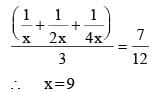∴ first number = 4x = 4 × 9 = 36
second number = 2x = 2 × 9 = 18
third number = x = 9

SSC CGL Previous Year Questions: Ratio, Proportion, Mixture and Partnership - 1 - Question 7

1/2 of A = 2/5 of B = 1/3 of C, then A : B : C is:    (SSC MTS 2017)

Detailed Solution for SSC CGL Previous Year Questions: Ratio, Proportion, Mixture and Partnership - 1 - Question 7

According to question,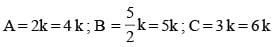∴ A : B : C = 4 : 5 : 6

SSC CGL Previous Year Questions: Ratio, Proportion, Mixture and Partnership - 1 - Question 8

The sum of the present ages of father and son is 90 years. 10 years earlier the ratio of their ages was 5 : 2. The present age of the father is:    (SSC MTS 2017)

Detailed Solution for SSC CGL Previous Year Questions: Ratio, Proportion, Mixture and Partnership - 1 - Question 8

Let present age of father = x
present age of son = y
According to question,
x + y = 90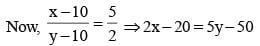⇒ 2x – 5y = –30 ...(ii)
from eq. (i) and (ii); x = 60 ; y = 30
∴ The present age of father = 60 years.

SSC CGL Previous Year Questions: Ratio, Proportion, Mixture and Partnership - 1 - Question 9

The third proportional of two numbers 9 and 24 is    (SSC CHSL 2017)

Detailed Solution for SSC CGL Previous Year Questions: Ratio, Proportion, Mixture and Partnership - 1 - Question 9

Here, a = 9, b = 24 and c = ?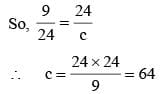SSC CGL Previous Year Questions: Ratio, Proportion, Mixture and Partnership - 1 - Question 10

If A : B = 2 : 5, B : C  = 4 : 3 and C : D = 2 : 1, then what is value of A : C : D?    (SSC CGL 2017)

Detailed Solution for SSC CGL Previous Year Questions: Ratio, Proportion, Mixture and Partnership - 1 - Question 10

A : B = 2 : 5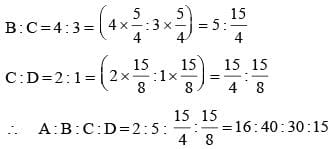∴ Value of A : C : D = 16 : 30 : 15

SSC CGL Previous Year Questions: Ratio, Proportion, Mixture and Partnership - 1 - Question 11

A, B and C invested amounts in the ratio 3 : 4 : 5 respectively. It the schemes offered compound interest at the rate of 20% per annum, 15% per annum and  10% per annum respectively, then what will be the ratio of their amounts after 1 year?    (SSC CGL 2017)

Detailed Solution for SSC CGL Previous Year Questions: Ratio, Proportion, Mixture and Partnership - 1 - Question 11

Let A, B, and C invested amounts in the ratio 300 : 400 : 500 respectively.
then,
∴ Required ratio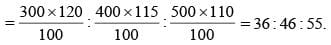SSC CGL Previous Year Questions: Ratio, Proportion, Mixture and Partnership - 1 - Question 12

₹ 3200 is divided among A, B and C in the ratio of 3 : 5 : 8 respectively. What is the difference (in ₹) between the share of B and C?    (SSC CGL 2017)

Detailed Solution for SSC CGL Previous Year Questions: Ratio, Proportion, Mixture and Partnership - 1 - Question 12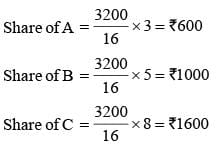∴ Difference between B and C = (1600 – 1000)  = ₹ 600.

SSC CGL Previous Year Questions: Ratio, Proportion, Mixture and Partnership - 1 - Question 13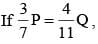then what is the ratio of P and Q respectively?    (SSC CGL 2017)

Detailed Solution for SSC CGL Previous Year Questions: Ratio, Proportion, Mixture and Partnership - 1 - Question 13

According to question,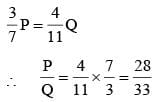SSC CGL Previous Year Questions: Ratio, Proportion, Mixture and Partnership - 1 - Question 14

The ratio of the radii of two cylinders is 2 : 1 and their heights are in the ratio 3 : 2. Then their volumes are in the ratio    (SSC Sub. Ins. 2016)

Detailed Solution for SSC CGL Previous Year Questions: Ratio, Proportion, Mixture and Partnership - 1 - Question 14

Ratio of volumes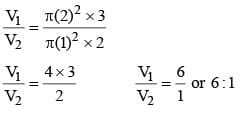SSC CGL Previous Year Questions: Ratio, Proportion, Mixture and Partnership - 1 - Question 15

A can is full of a mixture of a two liquids A and B in the ratio of 7 : 5. When 9 litres of mixture are drawn off from the can and replaced by the same quantity of liquid B, the ratio of A and B in the can becomes 7 : 9. The capacity of the can initially was    (SSC Sub. Ins. 2016)

Detailed Solution for SSC CGL Previous Year Questions: Ratio, Proportion, Mixture and Partnership - 1 - Question 15

Suppose, the can initially contains 7x and 5x of volume of a mixtures of A and B of volume respectively.
ATQ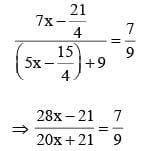⇒ 252 x – 189 = 140x + 147
⇒ 112x = 336 ⇒ x = 3
So, the can contained 21 litres of A initially.

SSC CGL Previous Year Questions: Ratio, Proportion, Mixture and Partnership - 1 - Question 16

The sum of the cubes of two numbers in the ratio 3 : 4 is 5824. The sum of the numbers is:    (SSC CGL 1st Sit. 2016)

Detailed Solution for SSC CGL Previous Year Questions: Ratio, Proportion, Mixture and Partnership - 1 - Question 16

Let the number be 3x and 4x
(3x)3 + (4x)3 =  5824
⇒ 27x3 + 64x3 =  5824 ⇒ 91x3 =  5824
⇒ x3 =  64 ⇒ x =  4
∴ Sum of numbers are = x(4 + 3) = 4× 7 = 28

SSC CGL Previous Year Questions: Ratio, Proportion, Mixture and Partnership - 1 - Question 17

The numbers x, y, z are respectively proportional to 2, 3, 5 and the sum of x, y and z is 80. If the number z is given by the equation z = ax – 8, then a is    (SSC CGL 1st Sit. 2016)

Detailed Solution for SSC CGL Previous Year Questions: Ratio, Proportion, Mixture and Partnership - 1 - Question 17

Let x, y and z be 2m, 3m and 5m.
According to Question,
2m + 3m + 5m = 80
⇒ 10m = 80 ⇒ m = 8
z = a, x – 8
∴ 5(8) = a(2)(8) – 8
⇒ 40 + 8 = 16 a ⇒ 48 = 16 a ⇒ a = 3

SSC CGL Previous Year Questions: Ratio, Proportion, Mixture and Partnership - 1 - Question 18

A and B invest ₹ 3000 and ₹ 2400 respectively in a business. If, after one year, there is a loss of ₹ 720, how much loss will B bear? (Loss or Profit is in proportion to their investments)    (SSC CGL 1st Sit. 2016)

Detailed Solution for SSC CGL Previous Year Questions: Ratio, Proportion, Mixture and Partnership - 1 - Question 18

A invested = ₹ 3000
B invested = ₹ 2400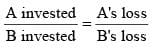According to Question,
(A + B) = ₹ 720
⇒ A = 720 – B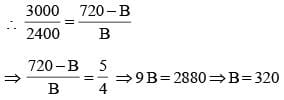SSC CGL Previous Year Questions: Ratio, Proportion, Mixture and Partnership - 1 - Question 19

The ratio of number of boys and girls in a school of 720 students is 7 : 5. How many more girls should be admitted to make the ratio 1 : 1?    (SSC CGL 1st Sit. 2016)

Detailed Solution for SSC CGL Previous Year Questions: Ratio, Proportion, Mixture and Partnership - 1 - Question 19

Ratio of boy and girl = 7 : 5
Number of student = 720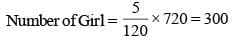Number of Boy = 720 – 300 = 420
Number of girl needed = 420 – 300 = 120

SSC CGL Previous Year Questions: Ratio, Proportion, Mixture and Partnership - 1 - Question 20

If A and B are in the ratio 4 : 5 and the difference of their squares is 81, what is the value of A?    (SSC CGL 1st Sit. 2015)

Detailed Solution for SSC CGL Previous Year Questions: Ratio, Proportion, Mixture and Partnership - 1 - Question 20

B2 - A2 = 81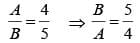Squaring both sides, we get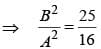both sides subtract 1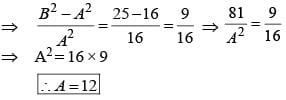Shortcut Method:
Let A be 4x and B be 5x
(5x)2 – (4x)2 = 81 (given)
9x2 = 81 ∴ x = 3
A = 3 × 4 = 12

## SSC CGL (Tier - 1) - Previous Year Papers (Topic Wise)

250 tests
Information about SSC CGL Previous Year Questions: Ratio, Proportion, Mixture and Partnership - 1 Page
In this test you can find the Exam questions for SSC CGL Previous Year Questions: Ratio, Proportion, Mixture and Partnership - 1 solved & explained in the simplest way possible. Besides giving Questions and answers for SSC CGL Previous Year Questions: Ratio, Proportion, Mixture and Partnership - 1, EduRev gives you an ample number of Online tests for practice

250 tests